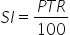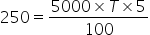Maths-
General
Easy

Question

# In how many years will a sum of Rs 5000 yield a simple interest of Rs 250 at the rate of 5% per annum?Hint:

## The correct answer is: Rs 250

### Complete step by step solution:We calculate simple interest by the formula,…(i)where P is Principal amount, T is number of years and R is rate of interestHere, we have R = 5%,SI = 250,P = 5000 and T = ?On substituting the known values in (i), we getHence, the sum of Rs 5000 earns a simple interest of Rs 250 in one year.#### With Turito Foundation.#### Get an Expert Advice From Turito.